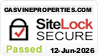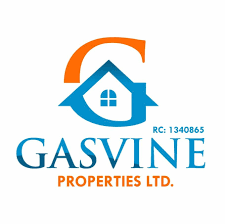24.2 C
Lagos
Saturday, September 23, 2023

## Making Housing Dreams A RealityDream Land Estate is located in  Ofada, Ogun State. About 4 minutes drive from the popular Yotomi Estate.

#### Features:

• Security
• Perimeter Fencing
• Drainage
• Light
• Swimming Pool

Outright or within 3 Months – =N=1,200,000

=N=120, 000 Monthly for 12 Months – =N=1,440,000

=N=70,000 Monthly for 24 Months – =N=1,680,000

=N=50,600 Monthly for 36 Monthly – =N=1,821,000

=N=42,917 Monthly for 48 Monthly – =N=2,060,000

HALF PLOT

Outright or Within 3 months – =N=600,000

=N=60,000 Monthly for 12 Months – =N=720,000

=N=35,000 Monthly for 24 Months – =N=840,000

=N=25,300 Monthly for 36 Months – =N=910,000

=N=21,459 Monthly for 48 Months – =N=1,030,000